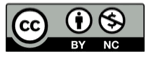# 利萨如图形的动态演示

2018-05-01## 动态演示

### 使用方法

• 两个振子的频率 $a, b$，比如 $a=2, b=3$
• 相位 $\theta, \delta$，比如 $\theta=\pi/2,\delta=0$

## 参数式

$$x(t) = \sin(at+\theta), y(t) = \sin(bt + \delta)$$

## 利萨如图形的性质

### 频率比

$a$ 与 $b$ 的比值 $a/b$ ，是决定利萨如图形状的首要因素， 强烈影响着利萨如图形的动态演示利萨如图形的形状。比如：

• 当 $a/b$ 为有理数时，图形曲线闭合
• 振动频率之比决定图形在$x$, $y$方向上的叶瓣的个数1:1直线
1:10°~90°椭圆
1:190°

## a:b=1:1$\phi= \pi/4$ 得到椭圆$\phi= \pi/2$ 得到圆（从参数式看出，$\sin$ 函数移相 $\pi/2$ 得到 $\cos$，于是图形是圆 ）（这一步是由两个振子得到圆，和 正弦函数 中、圆分解为两种振动的过程刚好相反）## a:b=1:2

$\phi=0$得到蝴蝶形状：$\phi = \pi/4$得到单根曲线：$\phi = \pi/2$又得到蝴蝶形状，不同的只是起始点位置：## 其他 a:b 值

$$a:b=1:3$$$$a:b=2:1$$$$a:b=5:4$$$$a:b=13:15$$## 精彩评论